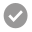Research Reports of the International Centre for Numerical Methods in Engineering (CIMNE)

##A stabilized Finite Element Method for analysis of fluid-structure interaction problems involving free surface waves

Abstract

A stabilized semi-implicit fractional step finite element method for solving coupled fluid-structure interaction problems involving free surface waves is presented. The stabilized equations are derived at a differential level via a finite element calculus procedure. A new mesh [...]

•351
•5/5
Julio García-Espinosa's Congress Presentations

##A finite element method for hydrodynamic testing of ship forms

Abstract

This paper presents advances in recent work of the authors to derive a fractional step scheme based on the stabilized finite element method that allows overcoming the above mentioned problem, resulting in a efficient time accurate scheme.

The starting point is the modified [...]

•33
Papers Repository of the International Centre for Numerical Methods in Engineering (CIMNE)

##The particle finite element method: a powerful tool to solve incompressible flows with free‐surfaces and breaking waves

Abstract

Particle Methods are those in which the problem is represented by a discrete number of particles. Each particle moves accordingly with its own mass and the external/internal forces applied [...]

•16
Papers Repository of the International Centre for Numerical Methods in Engineering (CIMNE)

##ODDLS: A new unstructured mesh finite element method for the analysis of free surface flow problems

Abstract

This paper introduces a new stabilized finite element method based on the finite calculus (Comput. Methods Appl. Mech. Eng.[...]

•28
Julio García-Espinosa's papers in WoS journals

##A finite element method for fluid-structure interaction with surface waves using a finite calculus formulation

Abstract

A stabilized semi-implicit fractional step finite element method (FEM) for solving coupled fluid-structure interaction problems involving free surface waves is presented. The stabilized governing equations for the viscous incompressible fluid and the [...]

•19
Julio García-Espinosa's papers in WoS journals

##An unstructured finite element solver for ship hydrodynamics problems

Abstract

A stabilized semi-implicit fractional step algorithm based on the ﬁnite element method for solving ship wave problems using unstructured meshes is presented. The stabilized gov-erning equations for the viscous incompressible ﬂuid and the free surface are derived at a differential [...]

•23
Papers Repository of the International Centre for Numerical Methods in Engineering (CIMNE)

##The Fixed‐Mesh ALE approach for the numerical simulation of floating solids

Abstract

In this paper, we propose a method to solve the problem of floating solids using always a background mesh for the spatial discretization of the fluid domain. The main feature of the method [...]

•1
Papers Repository of the International Centre for Numerical Methods in Engineering (CIMNE)

##An adaptive Fixed-Mesh ALE method for free surface flows

Abstract

In this work we present a Fixed-Mesh ALE method for the numerical simulation of free surface flows capable of using an adaptive finite element mesh covering a background [...]

•1
Revista Internacional de Métodos Numéricos para Cálculo y Diseño en Ingeniería

##A Lagrangian PFEM approach fornon-Newtonian viscoplastic materials

Abstract

This paper presents the application of a stabilized mixed Particle Finite Element Method (PFEM) to the solution of viscoplastic non-Newtonian flows. The application of the proposed model to the deformation of granular non-cohesive material is analysed. A variable yield threshold [...]

•34
Papers Repository of the International Centre for Numerical Methods in Engineering (CIMNE)

##An unstructured grid-based, parallel free surface solver

Abstract

An unstructured grid-based, parallel-free surface solver is presented. The overall scheme combines a finite-element, equal-order, projection-type 3-D incompressible flow solver with a finite [...]

•2